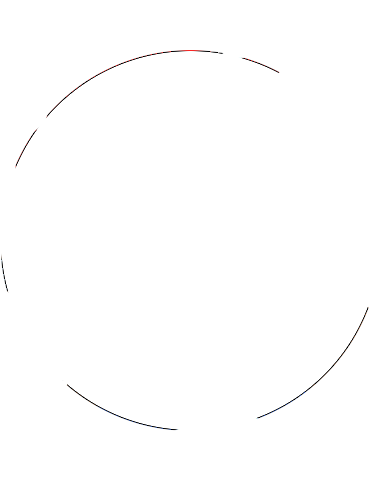# diffimg

Get the % difference in images using PIL's histogram + generate a diff image. Images should be the same size and have the same color channels (for example, RGB vs RGBA).### Installation

Now available from PyPi: `pip install diffimg`

### Usage

``````>>> from diffimg import diff
>>> diff('mario-circle-cs.png', 'mario-circle-node.png')
0.007319618135968298
``````

The very simple `diff` function returns a raw ratio instead of a % by default.

``````diff(im1_file,
im2_file,
delete_diff_file=False,
diff_img_file=DIFF_IMG_FILE
ignore_alpha=False)
``````

`im1_file, im2_file`: filenames of images to diff.

`delete_diff_file`: a fill showing the differing areas of the two images is generated in order to measure the diff ratio. Setting this to `True` removes it after calculating the ratio.

`diff_img_file`: filename for the diff image file. Defaults to `diff_img.png` (regardless of inputed file's types).

`ignore_alpha`: ignore the alpha channel for the ratio and if applicable, sets the alpha of the diff image to fully opaque.

### As command line tool

`python -m diffimg image1 image2 [-r/--ratio] [-d/--delete] [-f/--filename DIFF_IMG_FILE]`

`--ratio` outputs a number between 0 and 1 instead of the default `Images differ by X%`.

`--delete` removes the diff file after retrieving ratio/percentage.

`--filename` specifies a filename to save the diff image under. Must use a valid extension.

`--ignore-alpha` ignore the alpha channel.

### Tests

``````\$ ./test.py
......
----------------------------------------------------------------------
Ran 6 tests in 0.320s

OK
``````

### Formula

The difference is defined by the average % difference between each of the channels (R,G,B,A?) at each pair of pixels Axy, Bxy at the same coordinates in each of the two images (why they need to be the same size), averaged over all pairs of pixels.

For example, compare two 1x1 images A and B (a trivial example, >1 pixels would have another step to find the average of all pixels):

A1,1 = RGB(255,0,0) (pure red)

B1,1 = RGB(100,0,0) (dark red)

((255-100)/255 + (0/0)/255 + (0/0)/255))/3 = (155/255)/3 = 0.202614379

So these two 1x1 images differ by 20.2614379% according to this formula.

## Sample image 1## Sample image 2## Resulting diff image## Difference percentage output

`Images differ by 0.731961813597%`

Get A Weekly Email With Trending Projects For These Topics
No Spam. Unsubscribe easily at any time.
python (48,451
image-processing (469
testing-tools (188
diffing (20